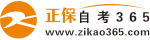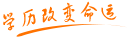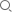#24小时客服：010-82335555# 自考“C语言程序设计”模拟试题一答案

2007年05月28日    来源：   字体：   打印

一、填空题

（1）整型、实型、字符型。

（2）int，2，-32768~ +32767

（3）1111111111011101

（4）- 112

（5）14，‘m’

（6）“a”是字符串常量，而‘a’是字符常量。

（7）存放地址值的变量或常量，或者答地址。

（8）取运算对象的地址。 取指针变量所指的内容。a=1，b=3，c=2

二、选择题

1、C     2、C     3、D     4、B      5、D

6、A     7、C     8、C     9、D      10、A

11、C    12、C    13、D    14、C     15、B

16、C    17、D    18、D    19、C     20、B

三、阅读程序题

（1） 3.141593，3.1416，3.142

（2） 6，8

（3） 4

（4） 22

（5） 19

四、程序填空题

1、scanf（“%f”，&f）；    sum+=f；

2、fopen（“myfile”，w）    fclose（fp）；

3、x>=0.0      x<=amin

五、编程题

1、main（）

{int a，b，c；

printf（“请输入三个整数：”）；

scanf（“%d，%d，%d”，&a，&b，&c）；

if（a<b）

if（b<c）    printf（“max=%d\n”，c）；

else      printf（“max=%d\n”，b）；

else if（a<c）  printf（“max=%d\n”，c）；

else    printf（“max=%d\n”，a）；}

2、#include“stdio.h”

main（）

{int I，n；

for（n=0，I=1900；I<=2000；I++）

{if（I%4==0&&I%100！=0||I%400==0）

{printf（“%d  ”，I）；n++；}

if（n%3==0）

printf（“\n”）；}  }   }

3、#include“stdio.h”

int fun（int a）

{int I；

if（a==2）     return 1；    I=2；

while（（a%I）！=0&&I<=sqrt（（float）a））

I++；

if（a%I==0）

{printf（“%d is not a prime！”，a）；

return 0；}

printf（“%d is a prime！”，a）；

return 1；  }

main（）

{int x；

scanf（“%d”，&x）；

printf（“%d\n”，fun（x））；  }

4、#include“stdio.h”

#define N 3

struct student

{long stu_num；      char sex；

char name；      int score；

float average；   }；

main（）

{int I，j，index；     float sum，max；

struct student stuarr[N]；

for（I=0；I<N；I++）

scanf（“%ld，%c，%s”，&stuarr[I].stu_num，&stuarr[I].sex，stuarr[I].name）；

for（j=0；j<4；j++）

scanf（“%d”，&stuarr[I].score[j]）； }

for（I=0；I<N；I++）

{sum=0.0；

for（j=0；j<4；j++）

sum+=stuarr[I].score[j]；

stuarr[I].average=sum/4.0；}

max=stuarr.average；

index=0；

for（I=1；I<N；I++）

if（stuarr[I].average>max）

{max=stuarr[I].average；

index=I；  }

printf（“总平均成绩最高的学生信息是学号=%ld，性别=%c，姓名=%s，平均成绩=%4.1f\n”，stuarr[index].stu_num，stuarr[index].sex，stuarr[index].name，stuarr[index].average）；

}